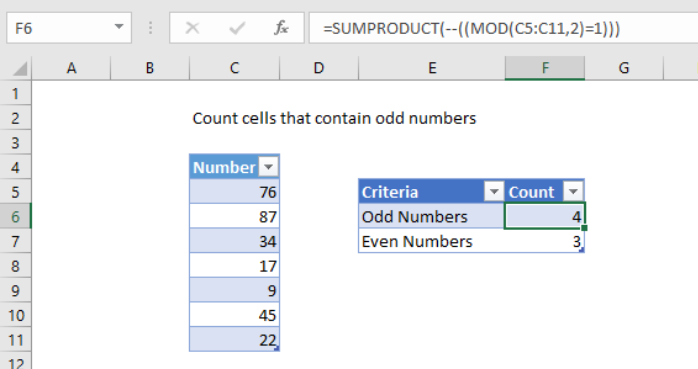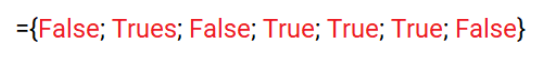Get instant live expert help with Excel or Google Sheets“My Excelchat expert helped me in less than 20 minutes, saving me what would have been 5 hours of work!”

#### Post your problem and you’ll get Expert help in seconds.

Your message must be at least 40 characters
Our professional Expert are available now. Your privacy is guaranteed.

# How to Count Cells That Contain Odd Numbers in Excel

Want to learn more about how to count cells that contain odd numbers using an Excel formula? This post will give you an overview of how to use the SUMPRODUCT formula to count those cells that contain odd numbers.

### Syntax(Generic Formula) to Count Cells Using Odd Numbers

`=SUMPRODUCT(--((MOD(Range,2)=1)))`

The MOD function in a formula returns the remainder after performing a division operation. The divisor in this division formula is 2, so the MOD function will generate a remainder of one(1) for an odd value and will return a remainder of zero(0) for any even numbers.

The range of cells which you want to count. The double negative {–} sign converts this array into a series of 1 and 0 values.

### Example and how the SUMPRODUCT works

The SUMPRODUCT function is used to multiply the corresponding components in two given arrays and returns the sum of those products. It returns the remainder when the number is divided by the divisor. The result has the same sign as divisor.Figure 1: Example of count all cells that contain odd numbers

To count all cells that contain odd numbers, you can use the SUMPRODUCT function. In the example selected cell shown, the formula in F6 is:

`=SUMPRODUCT(--((MOD(C5:C11,2)=1)))`

### Explanation

In the above example, the MOD(TestData,2)=0 function check whether the cell within the range containing the numbers are divisible by 2 or not.

The result stores an array like the following:If you have a long list of data in Excel, using this method will allow you to easily count the number of cells which contain odd numbers.

Still need some help with Excel formatting or have other questions about Excel? Connect with a live Excel expert here for some 1 on 1 help. Your first session is always free.

### Did this post not answer your question? Get a solution from connecting with the expert.Another blog reader asked this question today on Excelchat:
Solution examplesi have a table with Dates, First names , Last names and i want to count how many times a name occurs, but if a name occurs more than once on a particular date i need to only count it once. this is on excel and not google sheets
Solved by F. H. in 31 minsI need cell B2 Green if cells G2:Q2 are empty. If cells G2:Q2 have any text in any cell then i need B2 to be red.
Solved by C. W. in 20 minsI need help with this formula. I need this to include months =INT((M4)/7)&" weeks"&IF(MOD(M4,7)=0,"",", "&INT(MOD(M4,7))&" days") I have the number of Days to Start with
Solved by F. A. in 29 minsHi - I need a formula that can look at a Member Number (Column A) and report back if location (Column B) matches 1 of 6 locations, then looks to the date of occurance (Column N) but only adds back 1 value of repeating dates. I have this, but it is not coming back with the right answer... =SUMPRODUCT(('2017'!I\$3:I\$10000=\$A223)*ISNUMBER(SEARCH({"2","6","58","69","76","97"},'2017'!N\$3:N\$10000))) Information is corporate private, so I won't be uploading file.
Solved by S. L. in 60 minshi, i have this formula =SUMPRODUCT((B4:B464<>"")/COUNTIF(B4:B464,B4:B464&"")), i want to add a subtotal to it. so when I filter the column it also sums up the unique number if enteries i have filtered on.
Solved by F. L. in 60 mins## Subscribe to Excelchat.coAnother blog reader asked this question today on Excelchat: